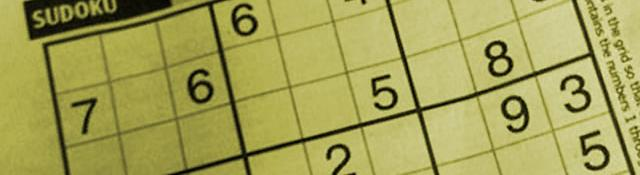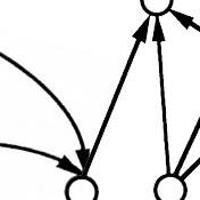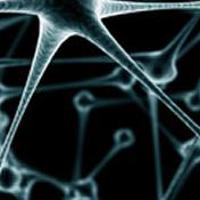## Extracting digits

Series: SuDoKu Grabber in OpenCV:

1. The Plot
2. Grid detection
3. Extracting the grid
4. Extracting digits
5. Recognizing digits

Okay, so we've detected the SuDoKu grid in the previous parts. Now, we'll try and recognize the digits already present in the image. To do that, we'll use a simple recognition technique: the k-Nearest Neighbors algorithm.

## The Digit Recognition Class

We'll create a new class to that handles all digit recognition. Start by creating a new .h and a .cpp file. In my case, I've named them as digitrecognizer.h and digitrecognizer.cpp. Add lines to digitrecognizer.h:

```#include <cv.h>
#include <highgui.h>

#include <ml.h>

using namespace cv;
#define MAX_NUM_IMAGES    60000
class DigitRecognizer
{
public:
DigitRecognizer();

~DigitRecognizer();

bool train(char* trainPath, char* labelsPath);

int classify(Mat img);

private:
Mat preprocessImage(Mat img);

private:
KNearest    *knn;
int numRows, numCols, numImages;

};
```

We've created a constructor and a destructor the class. The train() function takes a path to a dataset of images and a path to its corresponding labels. It will be similar to what was had in k-Nearest Neighbors for OpenCV.

classify() takens an image and returns what digit it is. preprocessImage() does some preprocessing... contrast enhancement, centering, etc. readFlippedInteger() is again similar to what was done in k-Nearest Neighbors for OpenCV. It has something to do with the endiannesss of your processor and the file-format of the dataset we're using.

knn is a k-Nearest Neighbor data structure... and numRows, numCols and numImages stores the number of rows and columns in the training dataset. numImages stores the number of images in the dataset.

## Defining the class

Open digitrecognizer.cpp and add these lines:

```DigitRecognizer::DigitRecognizer()
{
knn = new KNearest();

}

DigitRecognizer::~DigitRecognizer()
{
delete knn;
}
```

When you create a new instance of this class, you allocate memory for the k-Nearest Neighbor data structure. When you delete this class, you also delete the knn's memory.

```int DigitRecognizer::readFlippedInteger(FILE *fp)
{
int ret = 0;

BYTE *temp;

temp = (BYTE*)(&ret);

return ret;

}

bool DigitRecognizer::train(char *trainPath, char *labelsPath)
{
FILE *fp = fopen(trainPath, "rb");

FILE *fp2 = fopen(labelsPath, "rb");

if(!fp || !fp2)

return false;

// Read bytes in flipped order

fseek(fp2, 0x08, SEEK_SET);

if(numImages > MAX_NUM_IMAGES) numImages = MAX_NUM_IMAGES;

//////////////////////////////////////////////////////////////////
// Go through each training data entry and save a

// label for each digit

int size = numRows*numCols;

CvMat *trainingVectors = cvCreateMat(numImages, size, CV_32FC1);

CvMat *trainingClasses = cvCreateMat(numImages, 1, CV_32FC1);

memset(trainingClasses->data.ptr, 0, sizeof(float)*numImages);

BYTE *temp = new BYTE[size];
BYTE tempClass=0;
for(int i=0;i<numImages;i++)
{

trainingClasses->data.fl[i] = tempClass;

for(int k=0;k<size;k++)
trainingVectors->data.fl[i*size+k] = temp[k]; ///sumofsquares;

}

knn->train(trainingVectors, trainingClasses);
fclose(fp);

fclose(fp2);

return true;
}
```

For this series, I'll use the MNIST dataset of handwritten digits. I've used the data format used by them to read the file. Have a look at k-Nearest Neighbors for OpenCV for a more detailed explanation about how the above code works.

Now, for the very simple function: classify()!

```int DigitRecognizer::classify(cv::Mat img)
{
Mat cloneImg = preprocessImage(img);
return knn->find_nearest(Mat_<float>(cloneImg), 1);
}
```

This simply creates a clone preprocessed image. This image is sent into the k-Nearest Neighbor structure for recognition. Now we'll handle the preprocessing.

## Preprocessing images

Sending images directly to k-Nearest Neighbors is not a good idea. A little bit of work on the image can increase accuracy. Here, we'll center the actual digit in the image.Why? Because the MNIST dataset has been made in such a way - all digits are centered by their bounding box.

After centering, we'll fill things around the edges with black. This will eliminate any extra noise.

We'll divide the task into three parts:

1. Find approximate bounding box of the digit
2. Center the contents inside the bounding box
3. Flood fill edges with black color

### Finding the approximate bounding box

The idea is to start at the center of the image and move in all four directions. So you'll go up from the center, to the right, to the left and towards the bottom.

Lets say, you just moved one pixel up, towards the top of the image. You'll calculate the sum of all pixels in that row. If this sum is less than some value, you assume you've reached the top boundary. Otherwise, you keep moving on.

We do a similar summation for bottom, left and right. For left and right, you sum a column instead of a row. Here's the code that does this:

```Mat DigitRecognizer::preprocessImage(Mat img)
{

int rowTop=-1, rowBottom=-1, colLeft=-1, colRight=-1;

Mat temp;
int thresholdBottom = 50;
int thresholdTop = 50;
int thresholdLeft = 50;
int thresholdRight = 50;
int center = img.rows/2;
for(int i=center;i<img.rows;i++)
{
if(rowBottom==-1)
{
temp = img.row(i);
IplImage stub = temp;
if(cvSum(&stub).val < thresholdBottom || i==img.rows-1)
rowBottom = i;

}

if(rowTop==-1)
{
temp = img.row(img.rows-i);
IplImage stub = temp;
if(cvSum(&stub).val < thresholdTop || i==img.rows-1)
rowTop = img.rows-i;

}

if(colRight==-1)
{
temp = img.col(i);
IplImage stub = temp;
if(cvSum(&stub).val < thresholdRight|| i==img.cols-1)
colRight = i;

}

if(colLeft==-1)
{
temp = img.col(img.cols-i);
IplImage stub = temp;
if(cvSum(&stub).val < thresholdLeft|| i==img.cols-1)
colLeft = img.cols-i;
}
}
```

Startled? Let me explain. rowTop, rowBottom, colLeft and colRight mark the bounding box's top row, bottom row, etc. Initially, they're marked as -1 (representing no boundary was found) If the sum of a row/column is less than the corresponding threshold* then it is assumed to be the boundary.

Here, we're assuming all images have the same height and width. So we calculate a "center". The loop starts at "center" and goes till the number of rows.

Then, we check if the bottom boundary has been detected or not (based on -1). If now, a pointer to the i'th row is generated. Then we convert it into IplImage to use cvSum. (For some reason, there is no C++ version for this function on my system). If the sum is less than the threshold OR if we read the extreme bottom, we set the row bottom.

Each of the other three if- segments are similar, except for minor differences. For top, we use the (img.rows-i)th row (i goes from center-img.rows... but we need numbers in the reverse order). For left and right, we calculate sum over columns instead of rows.

### Center the bounding box's contents

Now, we know the size of the bounding box. We also know the size of the image. We can easily center the image:

```    Mat newImg;

newImg = newImg.zeros(img.rows, img.cols, CV_8UC1);

int startAtX = (newImg.cols/2)-(colRight-colLeft)/2;

int startAtY = (newImg.rows/2)-(rowBottom-rowTop)/2;

for(int y=startAtY;y<(newImg.rows/2)+(rowBottom-rowTop)/2;y++)
{
uchar *ptr = newImg.ptr<uchar>(y);
for(int x=startAtX;x<(newImg.cols/2)+(colRight-colLeft)/2;x++)
{
ptr[x] = img.at<uchar>(rowTop+(y-startAtY),colLeft+(x-startAtX));
}
}
```

We create a new image that's the same size as the original. Then, set startAtX to (the image's center x-width of bounding box/2). Similarly for set startAtY.

Then we simply read pixels from the original into newImg.

### Filling black along the edges

```    Mat cloneImg = Mat(numRows, numCols, CV_8UC1);

resize(newImg, cloneImg, Size(numCols, numRows));

// Now fill along the borders
for(int i=0;i<cloneImg.rows;i++)
{
floodFill(cloneImg, cvPoint(0, i), cvScalar(0,0,0));

floodFill(cloneImg, cvPoint(cloneImg.cols-1, i), cvScalar(0,0,0));

floodFill(cloneImg, cvPoint(i, 0), cvScalar(0));
floodFill(cloneImg, cvPoint(i, cloneImg.rows-1), cvScalar(0));
}
```

We're creating a new image. This time, the image is of the size of the training images (note the numCols, numRows). We iterate from 0 to the number of rows in cloneImg. And we flood fill black (0, 0, 0) on the top edge, bottom edge, right edge and the left edge.

### The final return

Finally, we reshape the numCols*numRows image into a single row image. The k-Nearest Neighbors algorithm expects images to be in this format.

```    cloneImg = cloneImg.reshape(1, 1);

return cloneImg;
}
```

The first 1 is the number of channels you want. The second 1 is for the number of rows you want.

## Summary

A long article. Have a look at the code, and try connecting it with your SuDoKu solver program.

## More in the series

This tutorial is part of a series called SuDoKu Grabber in OpenCV:

1. The Plot
2. Grid detection
3. Extracting the grid
4. Extracting digits
5. Recognizing digits

#### Related posts#### 7 unique neural network architectures